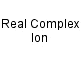[Co(H2O)6]2+
Cobalt(II) hexahydrated ion
Hexaaquacobolt(II) ionCo 1 H 12 O 6 Charge = +2

Real ion.

Gram formula weight (molecular mass) = 167.02

The hexaaquacobalt(III) ion is octahedral and is coloured pink.Hexaaquacobolt(II) ion
is defined with respect to the entities below:
The entities below are defined with respect to:
Hexaaquacobolt(II) ion
 Aqueous M(II) ion (AS/A2 generic)Complex (AS/A2 generic)Real complex ion (generic)Type 23 Lewis acid/base complex (generic)VSEPR geometry = AX6 (generic)Weak acid (AS/A2 generic)chemical compound molecule metal molecular science reaction mechanism ionic material acid base geometry reactivity synthesis science knowledge chemistry Lewis